# 深度学习（初识tensorflow2.版本）之三好学生成绩问题（1）

🔝🔝🔝🔝🔝🔝🔝🔝🔝🔝🔝🔝
🥰 博客首页：knighthood2001
😗 欢迎点赞👍评论🗨️
❤️ 热爱python，期待与大家一同进步成长！！❤️
👀 给大家推荐一款很火爆的刷题、面试求职网站 👀

# 三好学生成绩问题的引入

90 * w1 + 80 * w2 + 70 * w3 = 85

98 * w1 + 95 * w2 + 87 * w3 = 96

# 搭建解决三好学生成绩问题的神经网络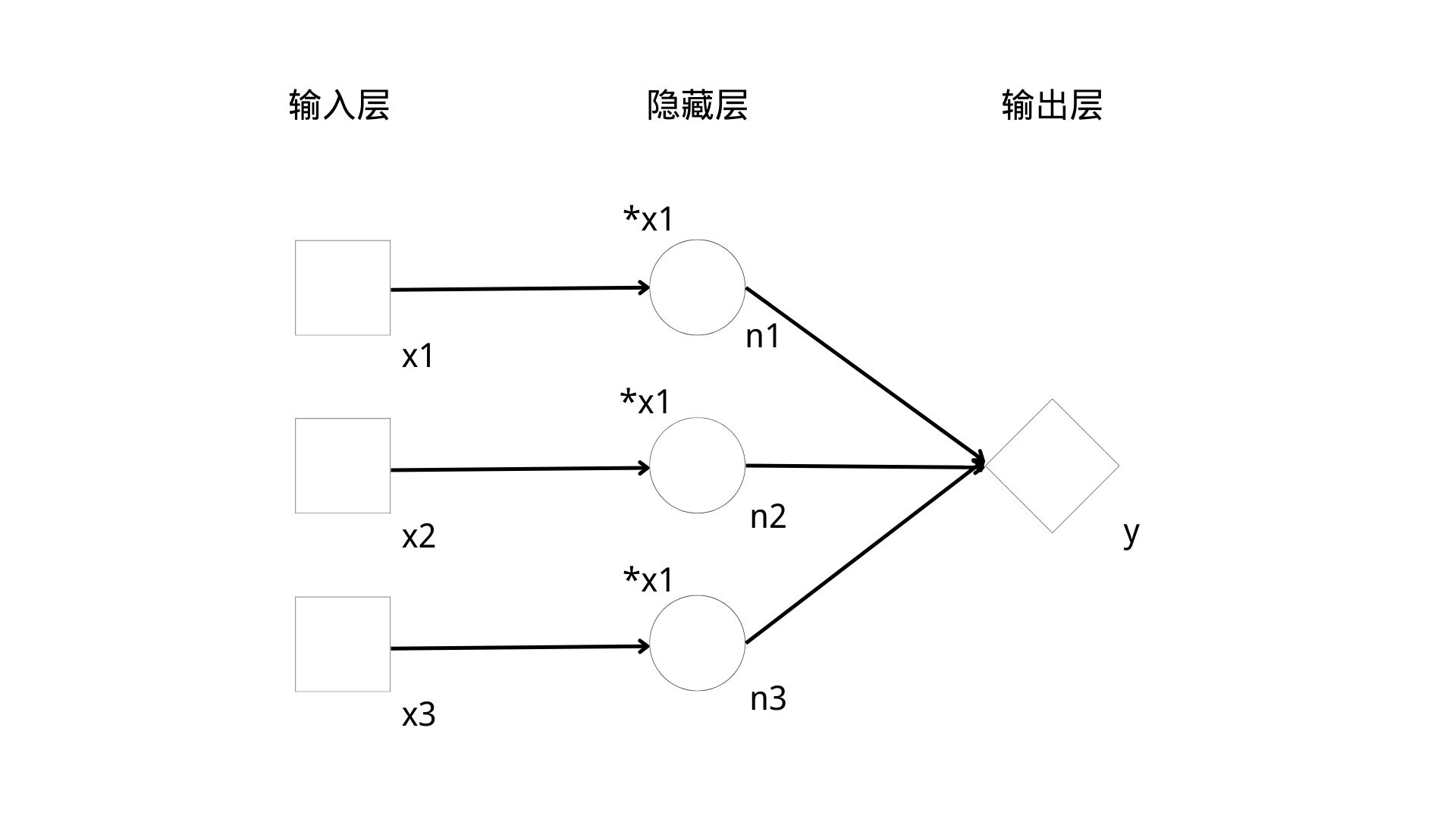②一般来说， 输入层是描述输入数据的形态的;我们用方块来代表每条输入数据的一个数 (或者叫一个字段)，叫作输入节点;输入节点一般用x来命名，如果有多个数值，则用x1，x2，...，xn来代表。

⑤我们在神经网络模型图中，一般约定在各个节点的 右下方(有时候因为拥挤也会在左下方)标记节点的名称，在节点的 左上方标记该节点所做的计算，例如，x1、x2、x3、n1、n2、n3、y都是节点名称，"w1"、 "w2"、 "*w3"这些都代表节点运算。

# 构建的神经网络的代码

``````import tensorflow as tf
# placeholder和eager execution不兼容
tf.compat.v1.disable_eager_execution()

# 定义三个输入节点
x1 = tf.compat.v1.placeholder(dtype=tf.float32)
x2 = tf.compat.v1.placeholder(dtype=tf.float32)
x3 = tf.compat.v1.placeholder(dtype=tf.float32)

# 定义权重（可变参数）
w1 = tf.Variable(0.1, dtype=tf.float32)
w2 = tf.Variable(0.1, dtype=tf.float32)
w3 = tf.Variable(0.1, dtype=tf.float32)

# 隐藏层
n1 = x1 * w1
n2 = x2 * w2
n3 = x3 * w3

# 输出层
y = n1 + n2 + n3

# 会话，管理神经网络运行的一个对象
sess = tf.compat.v1.Session()

init = tf.compat.v1.global_variables_initializer()

# 在sess会话中运行初始化这个函数
sess.run(init)

# 执行一次神经网络的计算
result = sess.run([x1, x2, x3, w1, w2, w3, y], feed_dict={x1: 90, x2: 80, x3: 70})
print(result)
# [array(90., dtype=float32), array(80., dtype=float32), array(70., dtype=float32), 0.1, 0.1, 0.1, 24.0]
``````

# 代码讲解

``````tf.compat.v1.disable_eager_execution()
``````

``````# 定义三个输入节点
x1 = tf.compat.v1.placeholder(dtype=tf.float32)
x2 = tf.compat.v1.placeholder(dtype=tf.float32)
x3 = tf.compat.v1.placeholder(dtype=tf.float32)
``````

dtype是"data type"的缩写，表示占位符所代表的数值的类型，tf.float32是tensorflow中的32位浮点数，即用32位二进制来代表一个小数，一般32位浮点数能满足计算的需要。

``````# 定义权重（可变参数）
w1 = tf.Variable(0.1, dtype=tf.float32)
w2 = tf.Variable(0.1, dtype=tf.float32)
w3 = tf.Variable(0.1, dtype=tf.float32)
``````

``````# 隐藏层
n1 = x1 * w1
n2 = x2 * w2
n3 = x3 * w3

# 输出层
y = n1 + n2 + n3
``````

[En]

The above completed the definition of the neural network model, and then take a look at how to input data in this neural network and get the operation results.

``````# 会话，管理神经网络运行的一个对象
sess = tf.compat.v1.Session()

init = tf.compat.v1.global_variables_initializer()

# 在sess会话中运行初始化这个函数
sess.run(init)
``````

``````# 执行一次神经网络的计算
result = sess.run([x1, x2, x3, w1, w2, w3, y], feed_dict={x1: 90, x2: 80, x3: 70})
print(result)
# [array(90., dtype=float32), array(80., dtype=float32), array(70., dtype=float32), 0.1, 0.1, 0.1, 24.0]
result = sess.run([x1, x2, x3, w1, w2, w3, y], feed_dict={x1: 98, x2: 95, x3: 87})
print(result)
# [array(98., dtype=float32), array(95., dtype=float32), array(87., dtype=float32), 0.1, 0.1, 0.1, 28.0]
``````

``````# [array(90., dtype=float32), array(80., dtype=float32), array(70., dtype=float32), 0.1, 0.1, 0.1, 24.0]
``````
``````# [array(98., dtype=float32), array(95., dtype=float32), array(87., dtype=float32), 0.1, 0.1, 0.1, 28.0]
``````

900.1+800.1+70*0.1=24

980.1+950.1+87*0.1=28

Original: https://blog.csdn.net/knighthood2001/article/details/125464728
Author: knighthood2001
Title: 深度学习（初识tensorflow2.版本）之三好学生成绩问题（1）

## Title: Pandas常用累计、同比、环比等统计方法实践案例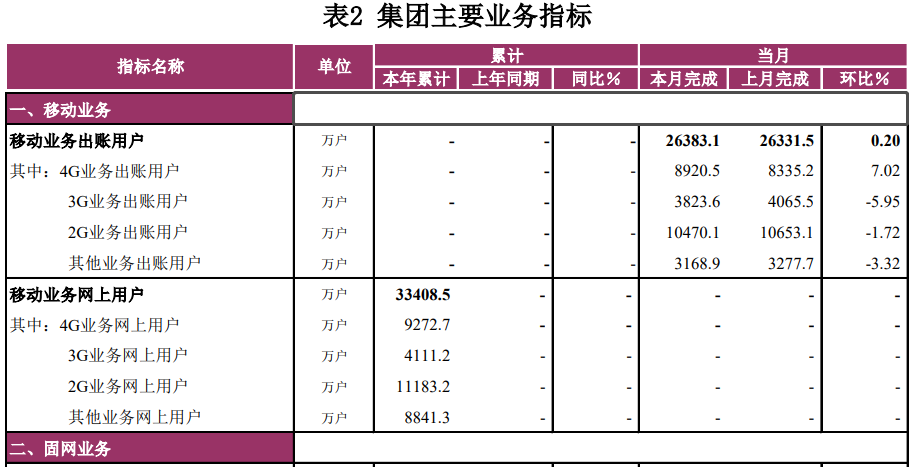• （本年）累计：是指本年1月到截止月份的合计数
• （上年）同期（累计）：是指去年1月到与本年累计所对应截止月份的合计数
• 同比（增长率）＝（本期数－同期数）/同期数*100％
• 环比（增长率）＝（本期数－上期数）/上期数*100％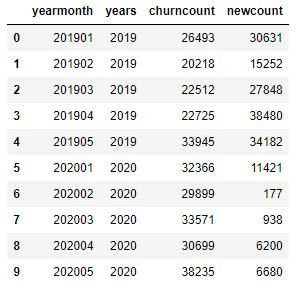# ; 1. （本年）累计

[En]

In the development of statistical analysis, it is quite common to accumulate some statistical data on an annual and monthly basis. For data, it is to accumulate data row by row according to the rules.

Pandas中的cumsum()函数可以实现按某时间维度累计需求。

``````
import pandas as pd

cum_columns_name = ['cum_churncount','cum_newcount']
df[cum_columns_name] = df[['years','churncount','newcount']].groupby(['years']).cumsum()
``````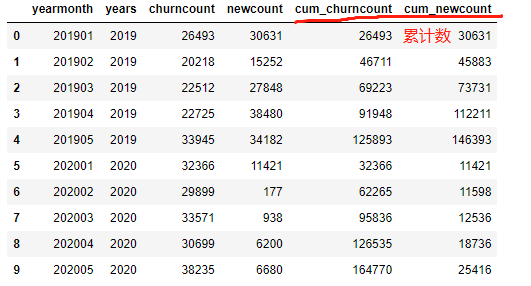# 2. （上年）同期累计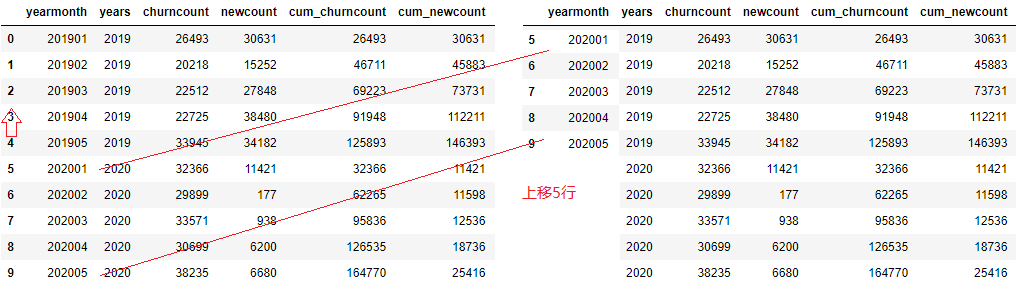``````cum_columns_dict = {'cum_churncount':'cum_same_period_churncount',
'cum_newcount':'cum_same_period_newcount'}
df_cum_same_period = df[['cum_churncount','cum_newcount','yearmonth']].copy()
df_cum_same_period = df_cum_same_period.rename(columns=cum_columns_dict)

df_cum_same_period.loc[:,'yearmonth'] = df_cum_same_period['yearmonth'].shift(-5)

df = pd.merge(left=df,right=df_cum_same_period,on='yearmonth',how='left')
``````

# 3. 上月（完成）

``````last_mnoth_columns_dict = {'churncount':'last_month_churncount',
'newcount':'last_month_newcount'}
df_last_month = df[['churncount','newcount','yearmonth']].copy()
df_last_month = df_last_month.rename(columns=last_mnoth_columns_dict)

df_last_month.loc[:,'yearmonth'] = df_last_month['yearmonth'].shift(-1)
df = pd.merge(left=df,right=df_last_month,on='yearmonth',how='left')

``````

# 4. 同比（增长率）

``````df.fillna(0,inplace=True)

df.loc[df['cum_same_period_churncount']!=0,'cum_churncount_rat'] = (df['cum_churncount']-df['cum_same_period_churncount'])/df['cum_same_period_churncount']
df.loc[df['cum_same_period_newcount']!=0,'cum_newcount_rat'] =  (df['cum_newcount']-df['cum_same_period_newcount'])/df['cum_same_period_newcount']
df[['yearmonth','cum_churncount','cum_newcount','cum_same_period_churncount','cum_same_period_newcount','cum_churncount_rat','cum_newcount_rat']]
``````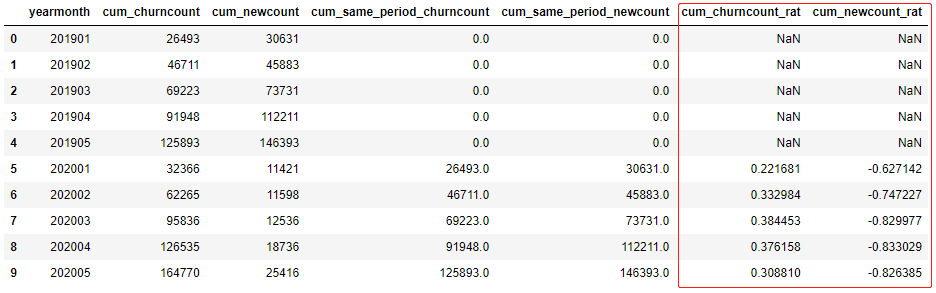# 5. 环比（增长率）

``````
df.loc[df['last_month_churncount']!=0,'churncount_rat'] = (df['churncount']-df['last_month_churncount'])/df['last_month_churncount']
df.loc[df['last_month_newcount']!=0,'newcount_rat'] =  (df['newcount']-df['last_month_newcount'])/df['last_month_newcount']
df[['yearmonth','churncount','newcount','last_month_churncount','last_month_newcount','churncount_rat','newcount_rat']]
``````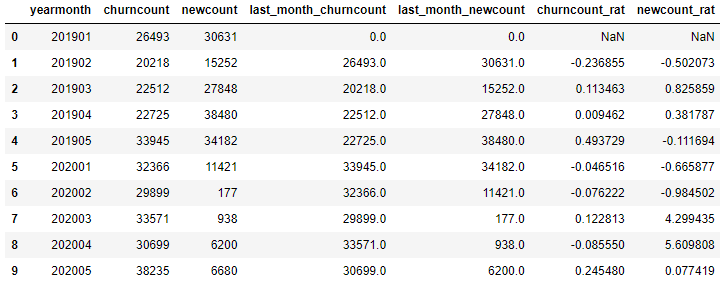# 6. 总结

pandas做统计计算功能方法比较多，这里总结用到的技术有累计cumsum()函数、移动数据shift()函数、表合并关联merge()函数，以及通过loc条件修改数据。

Original: https://blog.csdn.net/xiaoyw/article/details/122979421
Author: 肖永威
Title: Pandas常用累计、同比、环比等统计方法实践案例

## Yolov5更换backbone，与模型压缩（剪枝，量化，蒸馏）

~~~欢迎各位交流、star、fork、issues~~~ 项目介绍： 本仓库是基于官方yolov5源码的基础上，进行的改进。 目前支持更换yolov5的backbone主干网络为Ghostnet，以...

## python实现对语音信号的离散余弦变换（DCT）与离散余弦逆变换（IDCT）

python实现对语音的离散余弦变换与离散余弦逆变换 离散余弦变换 离散余弦逆变换 调包实现 离散余弦变换 离散余弦变换（DCT）信号谱分量丰富、能量集中，且不需要对语音相位进行估算等优点，在较低的运...